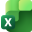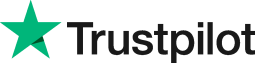Instructor-led training - 1 Day Advanced Excel Courses London
• Home
• Courses
• Promotions
• Schedule
• Formats
• Our Clients

#Excel Power Users Training Courses

Face to face / Virtual public schedule & onsite training. Restaurant lunch included at STL venues.

## Designed for Excel 365

 (113 reviews, see all 93,716 testimonials)

From £300 List price £350

Advanced Excel Training in London and UK Wide.

## Free manuals

We are providing a range of our course manuals free of charge.

## Training manual sample

Below are some extracts from our Excel training manuals.

### Excel Advanced: Formulas and Functions

#### What are Data Tables

Data Tables are often used by loan companies to show different loan amounts with repayments over various timescales. You may decide to use it when purchasing a car on finance, you can test your financial model with different assumptions about interest rates and down payments, and you can see the effects of varying rates on final costs you will pay, such as the total interest.

#### Using Data Tables

A data table summarises the impact of one or two variables on formulas that use those variables. You can click the What-if Analysis button in the Data Tools group on the Data tab and then click Data Table to create two kinds of data tables:

• Tables based on a single input variable that test the variable's impact on more than one formula
• Tables based on two input variables that test their impact on a single formula.

#### Data Tables Based on One Input Variable

Suppose you're considering buying a house that requires you to take on a 30-year, £200, 000 mortgage and you need to calculate monthly payments on the loan for several interest rates.
A one-variable data table, such as the one shown below, can give you the information you need.

To create this table, type the interest rates you want to test, as shown in cells B3:B9.
This is the input range, because it contains the input values you want to test.

Type the loan amount in a cell outside the data table area. We typed £200,000 in cell C1.
This allows us to easily change the loan amount to test various scenarios.

Enter the formula that uses the input variable. In this case, type the formula =PMT(A2/12, 360, C1) in cell C2. In this formula, A2/12 is the monthly interest rate, 360 is the term of the loan in months, and C1 refers to the cell containing the loan.

Note: Notice that the formula in cell C2 refers to cell A2, which is blank. This returns a zero value. Cell A2 is a placeholder through which Excel will feed the values in the input range to create the data table. Because Excel never changes the underlying value of this cell, this placeholder can be any cell, but it must be located outside the data table.

After you have entered the inputs and the formula:

• Select the data table-the smallest rectangular block that includes the formula and all the values in the input range.
• Select the range B2:C9
• Click the What-If Analysis button

Data Ribbon > Data Tools group > What If Analysis > Data Table

In the Data Table dialog box, shown below, specify the location of the input cell in the Row Input Cell or Column Input Cell box. The input cell is the placeholder cell referred to by the table formula-in this example, A2.

• If the input values are arranged in a row, type the input cell reference in the Row Input Cell box.
• If the values in the input range are arranged in a column

In our example, use the Column Input Cell box.

After you click OK, Excel enters the results of the table formula (one result for each input value) in the available cells of the data table range. In this example, Excel enters six results in the range C3:C9, as shown below, add currency formatting for easier reading.

When you create this data table, Excel enters the array formula { =TABLE(, A2)} in each cell in the results range C3:C9. In the sample data table, the formula computes the results of the PMT function using each of the interest rates in column B.
After you have built the table, you can change the loan amount or any of the interest rate values to see the results immediately.

Note: The TABLE function is an internal function, meaning that you can't select it in the Insert Function dialog box or type it manually.

#### Single-Variable Tables with More Than One Formula

When you create a single-variable data table, you can include as many output formulas as you want. If your input range is in a column, type the second output formula directly to the right of the first one, the third to the right of the second, and so on.
You can use different formulas for different columns, but they must all use the same input cell.

#### Mortgage Scenario

Suppose you're thinking about buying a house that would require you to take out a £180,000 mortgage. You want to know what your monthly payments would be on that mortgage at each of the interest rates in the input range, and you want to be able to compare these payments with those for the £200,000 mortgage calculated in our previous example. You can expand the table to include both formulas.

To add a new formula to the existing data table, type the new formula in cell D2. For this example, we typed =-PMT(A2/12, 360, D1), note the minus sign before the PMT.

This formula must also refer to cell A2, the same input cell as in the first formula. Then type £180,000 in cell D1, and select the table range B2:D9. Then click the What-If Analysis button on the Data tab, and click Data Table. Finally, type the same input cell reference (\$A\$2) in the Column Input Cell box.

Note: that we have included a minus (-) symbol before the PMT function. This will format the results without showing the minus sign as seen in the previous example in column C. This is optional.

#### Data Tables Based on Two Input Variables

Suppose you want to build a data table that computes the monthly payment on a £200,000 mortgage, but this time you want to vary not only the interest rate but also the term of the loan. You want to know what effect changing the interest rate and the term will have on your monthly payment.

To create this table, you can again type seven interest rates in cells B3: B9, and then type the second set of input values-the loan terms, in months-in a row above and to the right of the first set, as shown below.

After you type the loan amount in a cell outside the table area (cell I2 in this example), you can create the table formula. Because this is a two-variable table, you must type the formula in the cell at the intersection of the row and column that contain the two sets of input values-cell B2, in this example.

NOTE: Although you can include as many formulas as you want in a single-variable data table, you can include only one output formula in a two-variable table.

The formula for the table in this example is =-PMT(A2/12, B1, I2).

You'll notice immediately that the formula in cell B2 returns the #NUM! error value. This is because the two blank cells, A2 and B1, when used as arguments, produce a number that is either too large or too small for Excel to represent.

Finally, select the data table-the smallest rectangular block that includes all the input values and the table formula. In this example, the table range is B2:F9.

Click the What-If Analysis button on the Data tab, then click Data Table, and finally specify the (empty) input cells. Because this is a two-variable table, you must define two input cells.

For this example, type the reference for the first input cell, \$B\$1, in the Row Input Cell box, and then type the reference for the second input cell, \$A\$2, in the Column Input Cell box.

The final Data Table is formatted to Currency and columns widened. Remember the error shown in cell B2 in the above example does not affect the working of the table. If you prefer not to see this cell, change the font colour to white.

Be careful not to reverse the input cells in a two-variable table.

To make sure you're using the correct input cells, you need to look at the formula. In our example formula =-PMT(A2/12, B1, I2), A2 appears in the first argument, which is rate. Because the rates are arranged in a column, A2 is the column input cell.

#### Editing Data Tables

Although you can edit the input values or formulas in the left column or top row of a table, you can't edit the contents of any individual cell in the results range because the data table is an array. If you make a mistake when you set up a data table, you must select all the results, press the Delete key, and then refresh the table.

#### Copy Data Tables

You can copy the table results to a different part of the worksheet. You might want to do this to save the table's current results before you change the table formula or variables.

When you copy and paste the copied values are constants, not array formulas. Excel automatically changes the results of the table from a set of array formulas to their numeric values if you copy the results out of the table range; this is the equivalent of a Copy and Paste Value method.